#5 Reasons Logistic Regression should be the first thing you learn when becoming a Data Scientist

Learn Logistic Regression first to become familiar with the pipeline and not being overwhelmed with fancy algorithms.comments

By Dima Shulga, Data Scientist at HiredScoreI started my way in the Data Science world a few years back. I was a Software Engineer back then and I started to learn online first (before starting my Master’s degree). I remember that as I searched for online resources I saw only names of learning algorithms — Linear Regression, Support Vector Machine, Decision Tree, Random Forest, Neural Networks and so on. It was very hard to understand where I should start. Today I know that the most important thing to learn to become a Data Scientist is the pipeline, i.e, the process of getting and processing data, understanding the data, building the model, evaluating the results (both of the model and the data processing phase) and deployment. So as a TL;DR for this post: Learn Logistic Regression first to become familiar with the pipeline and not being overwhelmed with fancy algorithms.

You can read more about my experience of moving from Software Engineering into Data Science in this post.

So here’s my 5 reasons why today I think that we should start with Logistic Regression first to become a Data Scientist. This is only my opinion of course, for other people it might be easier to do things in a different way.

Because the learning algorithm is just a part of the pipeline

As I said in the beginning, the Data Science work is not just model building. It includes these steps: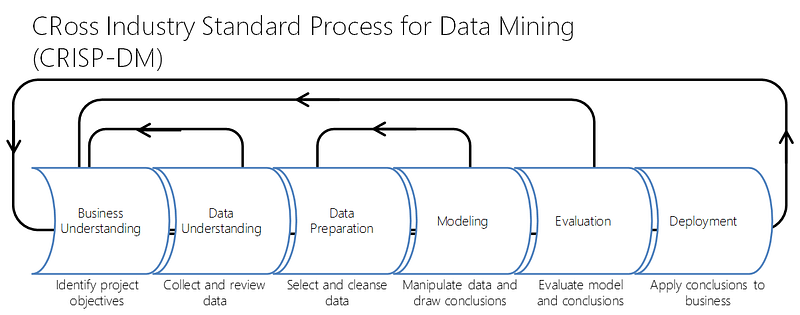You can see that “Modeling” is one part of this repetitive process. When building a data product, it is a good practice to build your whole pipeline first, keep it simple as possible, understand what exactly you’re trying to achieve, how can you measure yourself and what is your baseline. After that, you can do fancy Machine Learning and be able to know if you’re getting better.

By the way, Logistic Regression (or any ML algorithm) may be used not only in the “Modeling” part but also in “Data Understanding” and “Data Preparation”, imputing is one example for this.

Because you’ll better understand Machine Learning

I think that the first question people ask themselves when reading this post title is why “Logistic” and not “Linear” regression. And the truth is that it doesn’t matter. This question alone brings to notion 2 types of supervised learning algorithms — Classification (Logistic Regression) and Regression (Linear Regression). When you build your pipeline with Logistic or Linear Regression you’re becoming familiar with most of the Machine Learning concepts while keeping things simple. Concepts like Supervised and Unsupervised Learning, Classification vs Regression, Linear vs Non-Linear problems and many more. Also you’re getting an idea about how to prepare your data, what challenges might be there (like imputing and feature selection), how do you measure your model, should you use “Accuracy”, “Precision-Recall”, “ROC AUC”? or maybe “Mean Squared Error” and “Pearson Correlation”?. All those concepts are the most important part of the Data Science process. After you’re familiar with them, you’ll be able to replace your simple model with much more complex one onces you’ve mastered them.

Because “Logistic Regression” is (sometimes) enough

Logistic regression is a very powerful algorithm, even for very complex problems it may do a good job. Take MNIST for example, you can achieve 95% accuracy using Logistic Regression only, it’s not a great result, but its more than good enough to make sure you pipeline works. Actually, with the right representation of the features, it can do a fantastic job. When dealing with non-linear problems, we sometimes try to represent the original data in a way that may be explained linearly. Here’s a small example of this idea: We want to perform a simple classification task on the following data:

```X1    x2    |  Y
==================
-2    0        1
2    0        1
-1    0        0
1    0        0```

If we plot this data, we’ll be able to see that there is no single line that can separate it:

```plt.scatter([-2, 2], [0, 0 ], c='b')
plt.scatter([-1, 1], [0, 0 ], c='r')```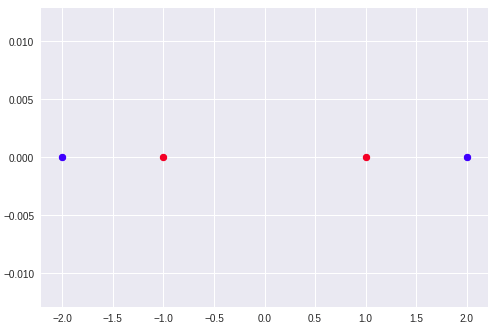In this case, Logistic Regression without doing something with the data won’t help us, but if we drop our `x2` feature and use `x1²` instead, it will look like this:

```X1    x1^2  |  Y
==================
-2    4       1
2    4       1
-1    1       0
1    1       0```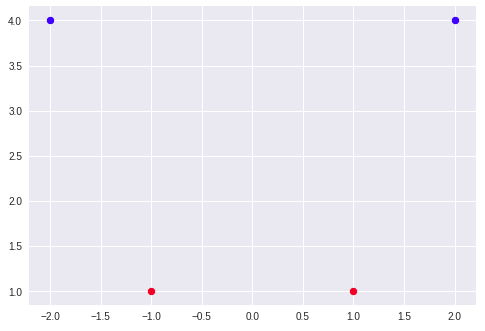Now, there is a simple line that can separate the data. Of course, this toy example is nothing like real life, and in real life, it will be very hard to tell how exactly you need to change your data so a linear classier will help you, but, if you invest some time in feature engineering and feature selection your Logistic Regression might do a very good job.

Because it is an important tool in Statistics

Linear Regression is good not only for prediction, once you have a fitted Linear Regression model you can learn things about relationships between the depended and the independent variables, or in more “ML” language, you can learn the relations between your features and you target value. Consider a simple example where we have data about house pricing, we have a bunch of features and the actual price. We fit a Linear Regression model and get good results. We can look at the actual weights the model learned for each feature, and if those are significant, we can say that some feature is more important than others, moreover, we can say that the house size, for example, responsible for 50% of the change in the house price and increase in 1 square meter will lead to increase in 10K in house price. Linear Regression is a powerful tool to learn relationships from data and statisticians use it quite often.

Because its a great start to learning Neural Networks

For me, studying Logistic regression first helped a lot when I started to learn Neural Networks. You can think of each neuron in the network as a Logistic Regression, it has the input, the weights, the bias you do a dot product to all of that, then apply some non linear function. Moreover, the final layer of a neural network is a simple linear model (most of the time). Take a look at this very basic neural network: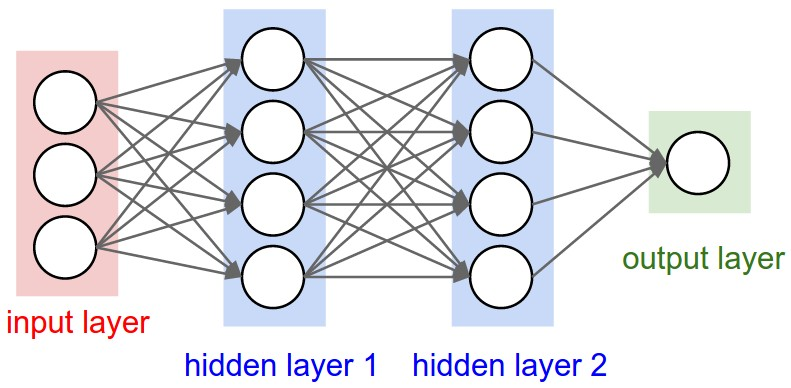Let’s look closer at the “output layer”, you can see that this is a simple linear (or logistic) regression, we have the input (hidden layer 2), we have the weighs, we do a dot product and then add a non linear function (depends on the task). A nice way to think about neural networks is dividing the NN into two parts, the representation part, and the classification/regression part: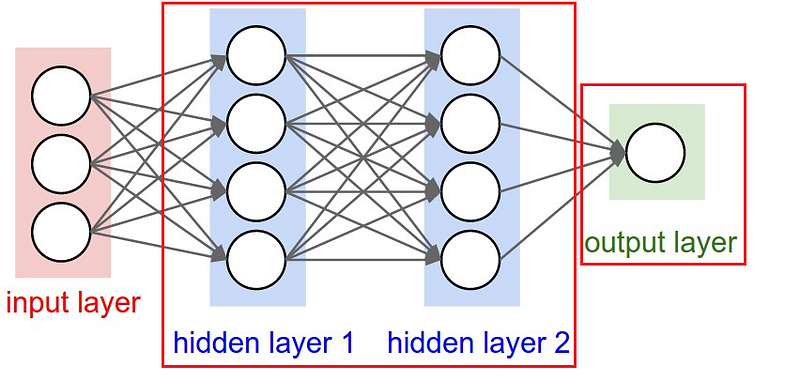The first part (on the left) is trying to learn a good representation of the data that will help the second part (on the right) to perform a linear classification/regression. You can read more about that idea in this great post.

Conclusion

There’s a lot to know if you want to become a Data Scientist, and at first glance, it looks like the learning algorithms are the most important part. The reality is that the learning algorithms are very complicated in most cases and require a lot of time and effort to understand, but are only a small part of the Data Science pipeline.

Bio: Dima Shulga is a Data Scientist at HiredScore.

Original. Reposted with permission.

Related:

By subscribing you accept KDnuggets Privacy Policy

By subscribing you accept KDnuggets Privacy Policy

By subscribing you accept KDnuggets Privacy Policy